# Write an algorithm that estimates the desired eigenvalues of an input matrix by using the power...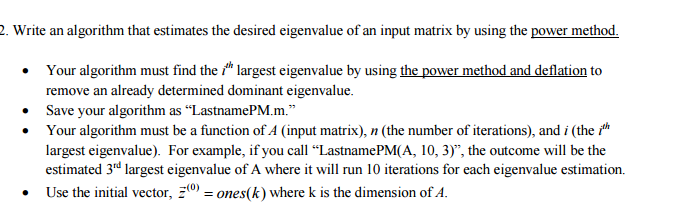Write an algorithm that estimates the desired eigenvalues of an input matrix by using the power method. Your algorithm must find the i^th largest eigenvalues by using the power method and deflation to remove an already determined dominant eigenvalues. Save your algorithm as "LastnamePM.m." Your algorithm must be a function of A (input matrix), n (the number of iterations), and i (the i^th largest eigenvalues). For example, if you call "LastnamePM(A, 10,3)", the outcome will be the estimated 3,d largest eigenvalues of A where it will run 10 iterations for each eigenvalues estimation. Use the initial vector, z^(0) = ones(k) where k is the dimension of A.

http://math.oregonstate.edu/~math_reu/proceedings/REU_Proceedings/Proceedings1993/1993MacLean.pdf

http://math.unice.fr/~frapetti/CorsoF/cours4part2.pdf

http://www.math.pitt.edu/~sussmanm/2071Spring09/lab08/

http://people.inf.ethz.ch/arbenz/ewp/Lnotes/chapter6.pdf

http://www.jmlr.org/papers/volume14/yuan13a/yuan13a.pdf

#### Earn Coin

Coins can be redeemed for fabulous gifts.

Similar Homework Help Questions
• ### Take any system of 3x3 matrix equation which is linear Solve the system using generic algorithm...

Take any system of 3x3 matrix equation which is linear Solve the system using generic algorithm for the Gauss Seidel method The code must take the matrix input by the user It should be solvable for any nxn system Give only a working proper MATLAB code if you dont know do not answer or i will dislike badly compare with original solution and then break.

• ### PLEASE USE MATLAB. The code is basically given to you with the algorithm above. Write a...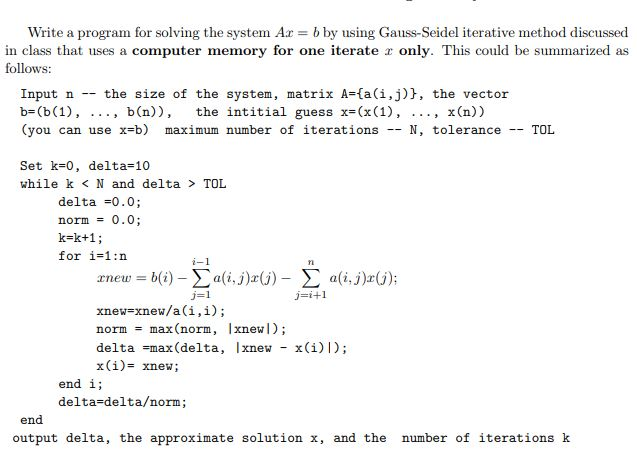PLEASE USE MATLAB. The code is basically given to you with the algorithm above. Write a program for solving the system Ar-b by using Gauss-Seidel iterative method discussed in class that uses a computer memory for one iterate only. This could be summarized as follows: Input n -- the size of the system, matrix A-(a(i,j), the vectoir b (b (1), . . . , b(n)), the intitial guess x (x(1), ..., x(n)) (you can use x=b) maximum number of iterations...

• ### NEED HELP WITH PROBLEM 1 AND 2 OF THIS LAB. I NEED TO PUT IT INTO...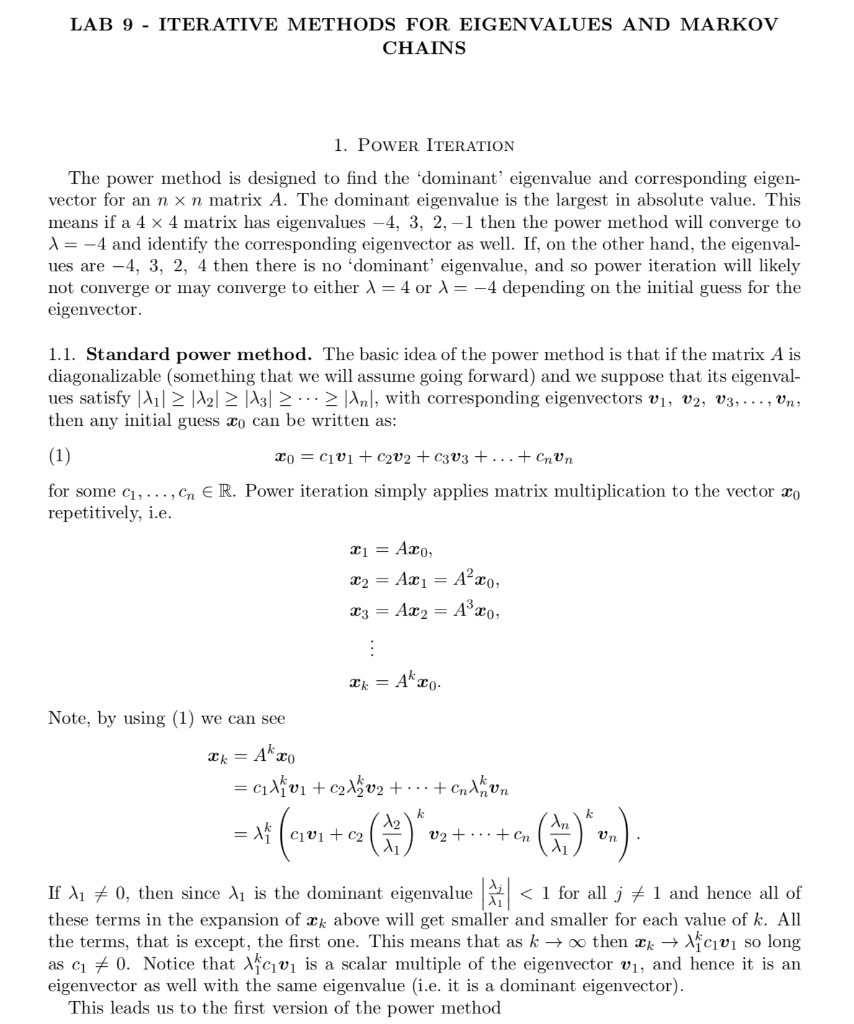NEED HELP WITH PROBLEM 1 AND 2 OF THIS LAB. I NEED TO PUT IT INTO PYTHON CODE! THANK YOU! LAB 9 - ITERATIVE METHODS FOR EIGENVALUES AND MARKOV CHAINS 1. POWER ITERATION The power method is designed to find the dominant' eigenvalue and corresponding eigen- vector for an n x n matrix A. The dominant eigenvalue is the largest in absolute value. This means if a 4 x 4 matrix has eigenvalues -4, 3, 2,-1 then the power method...

• ### 1. If the ax matrix A has eigenvalues ....., what are the eigenvalues of a) 4*,...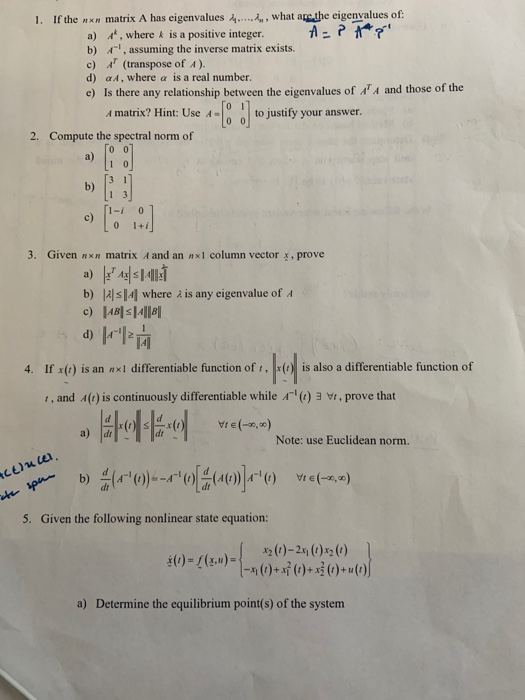1. If the ax matrix A has eigenvalues ....., what are the eigenvalues of a) 4*, where & is a positive integer. AE? A ' b) ', assuming the inverse matrix exists. c) A' (transpose of ). d) a, where a is a real number. e) Is there any relationship between the eigenvalues of 'A and those of the A matrix? Hint: Use to justify your answer. 2. Compute the spectral norm of 0 0 b) c) c) 1-1 0...

• ### In this exercise, you will work with a QR factorization of an mxn matrix. We will proceed in the ...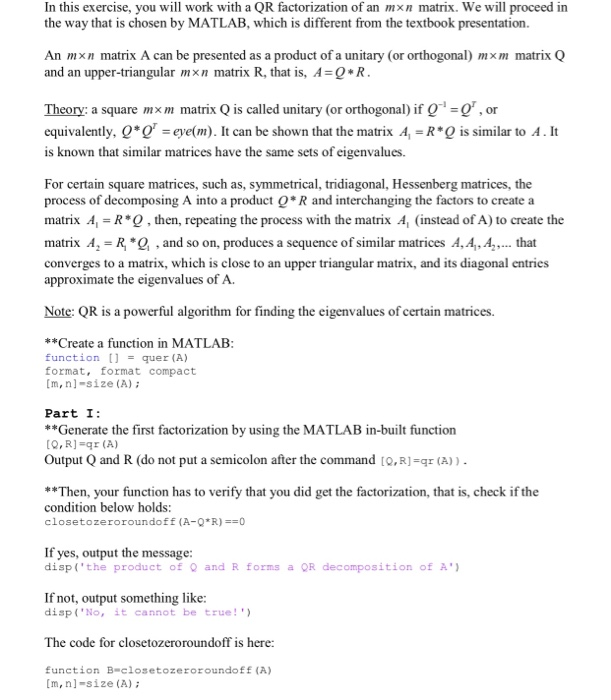In this exercise, you will work with a QR factorization of an mxn matrix. We will proceed in the way that is chosen by MATLAB, which is different from the textbook presentation. An mxn matrix A can be presented as a product of a unitary (or orthogonal) mxm matrix Q and an upper-triangular m × n matrix R, that is, A = Q * R . Theory: a square mxm matrix Q is called unitary (or orthogona) if -,or equivalently,...

• ### (a) Write the following function in Matlab [eval, evec] -power method (A, x, tol) The inputs...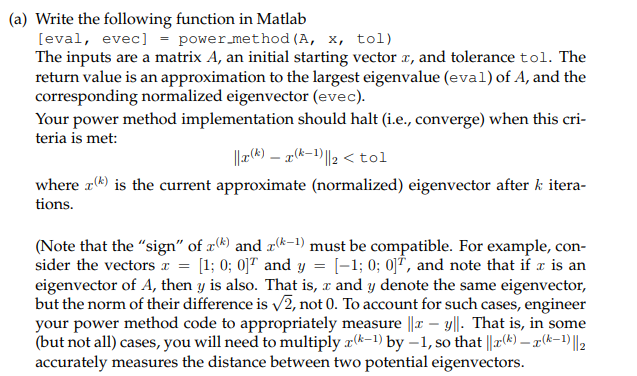(a) Write the following function in Matlab [eval, evec] -power method (A, x, tol) The inputs are a matrix A, an initial starting vector r, and tolerance tol. The return value is an approximation to the largest eigenvalue (eval) of A, and the corresponding normalized eigenvector (evec) Your power method implementation should halt (i.e., converge) when this cri- teria is met: where (k) is the current approximate (normalized) eigenvector after k itera- tions. (Note that the "sign" of r() and...

• ### please solve the Program USING MATLAB. do not do #2. write in details for the command...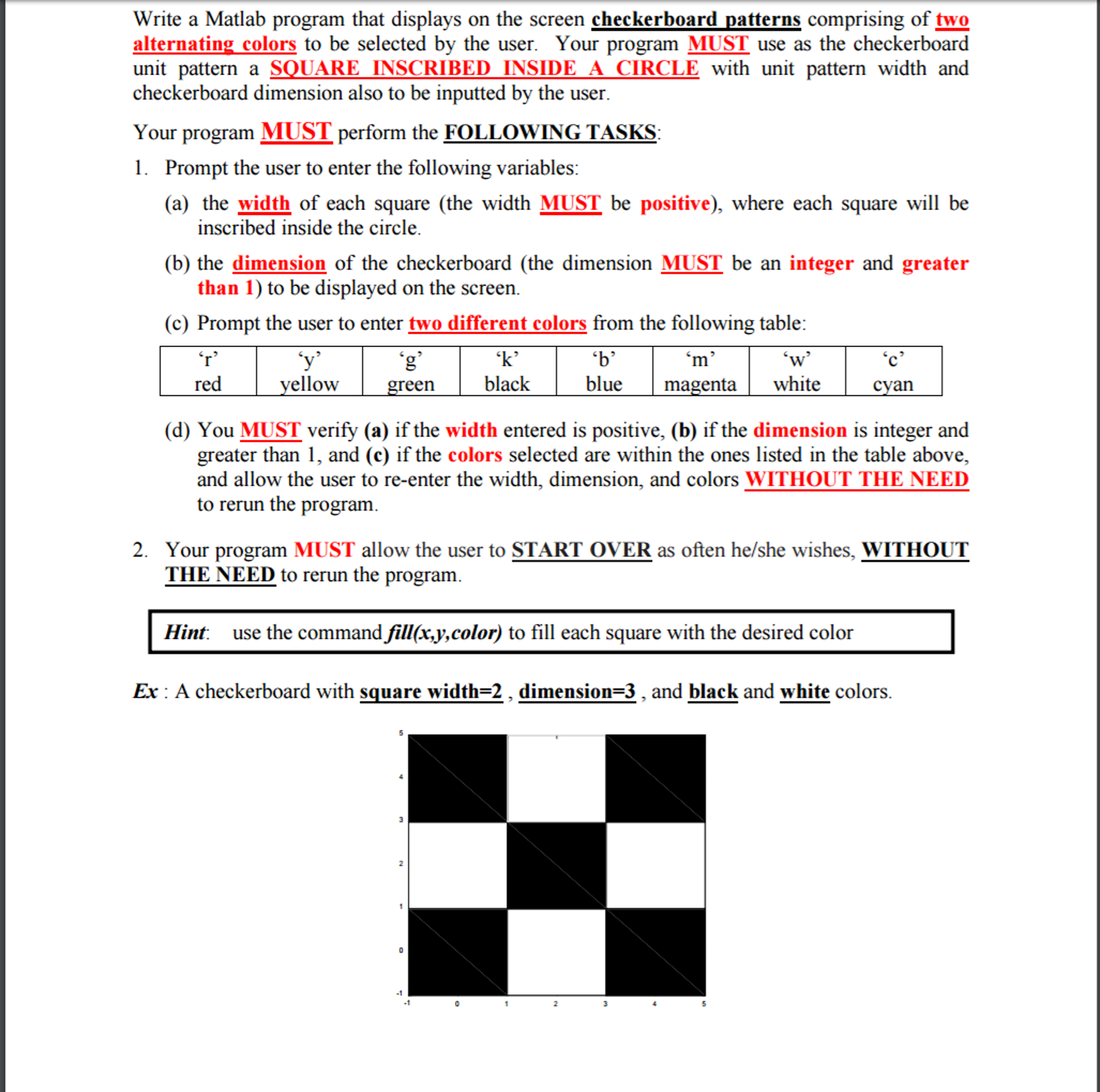please solve the Program USING MATLAB. do not do #2. write in details for the command lines. Make sure the program runs and also completely does what it askes please Write a Matlab program that displays on the screen checkerboard patterns comprising of two alternating_colors to be selected by the user. Your program MUST use as the checkerboard unit pattern a SQUARE INSCRIBED INSIDE A CIRCLE with unit pattern width and checkerboard dimension also to be inputted by the user....

• ### Can you help me with this question please? For the code, please do it on MATLAB. Thanks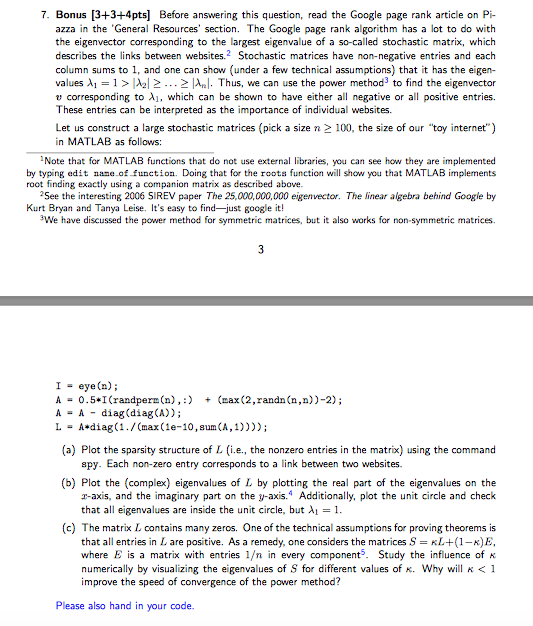Can you help me with this question please? For the code, please do it on MATLAB. Thanks 7. Bonus [3+3+4pts] Before answering this question, read the Google page rank article on Pi- azza in the 'General Resources' section. The Google page rank algorithm has a lot to do with the eigenvector corresponding to the largest eigenvalue of a so-called stochastic matrix, which describes the links between websites.2 Stochastic matrices have non-negative entries and each column sums to1, and one can...

• ### (b) . Write the k-th step of the trapezoidal method as a root-finding problem Ğ = is Y+1 where the unknown (e)Find the Jacobian matrix of the vector function from the previous part. (dWrite a funct...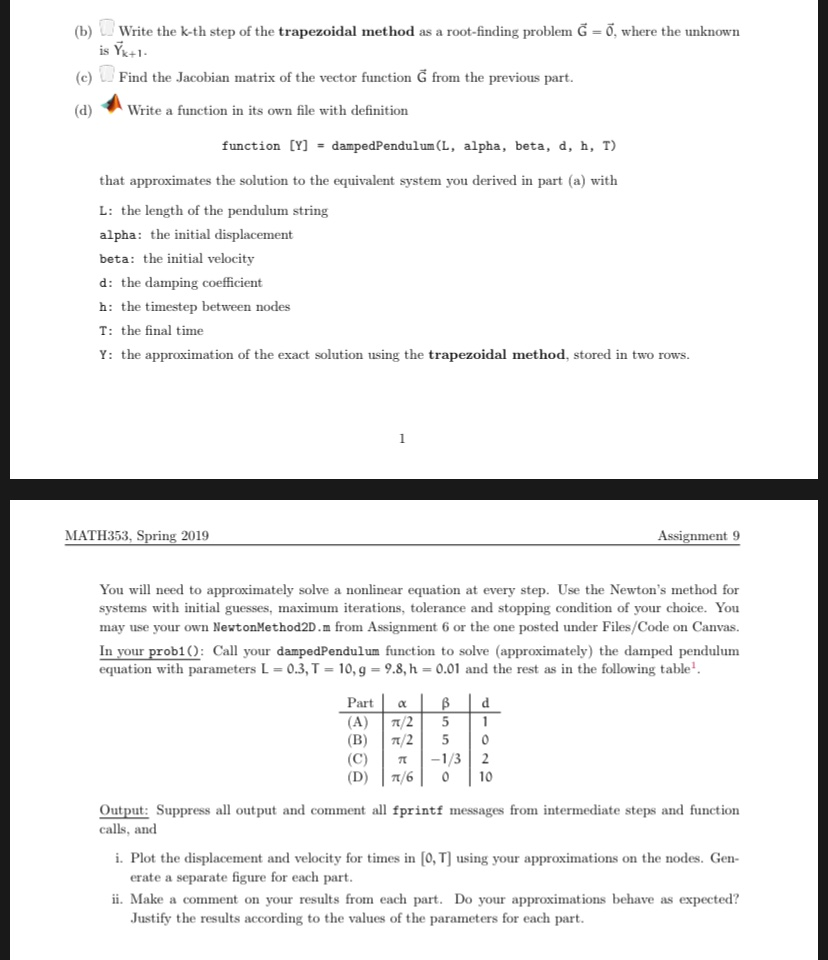(b) . Write the k-th step of the trapezoidal method as a root-finding problem Ğ = is Y+1 where the unknown (e)Find the Jacobian matrix of the vector function from the previous part. (dWrite a function in its own file with definition [Y] dampedPendulum(L, T) function alpha, beta, d, h, that approximates the solution to the equivalent system you derived in part (a) with L: the length of the pendulum string alpha: the initial displacement beta: the initial velocity d:...

• ### Refer to the following program sample to answer the parts (a-i) below. Keep in mind that low and high are both indices of array A. /1 Assume that A is a sorted array containing N integers // Assume t...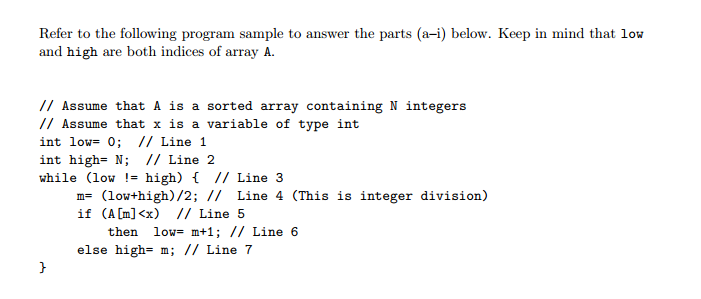Refer to the following program sample to answer the parts (a-i) below. Keep in mind that low and high are both indices of array A. /1 Assume that A is a sorted array containing N integers // Assume that x is a variable of type int int low= 0; // Line 1 int high- N; // Line 2 while (low- high) I/ Line 3 m- (lowthigh)/2; // Line 4 (This is integer division) if (A [m]<) I/Line 5 then low-...